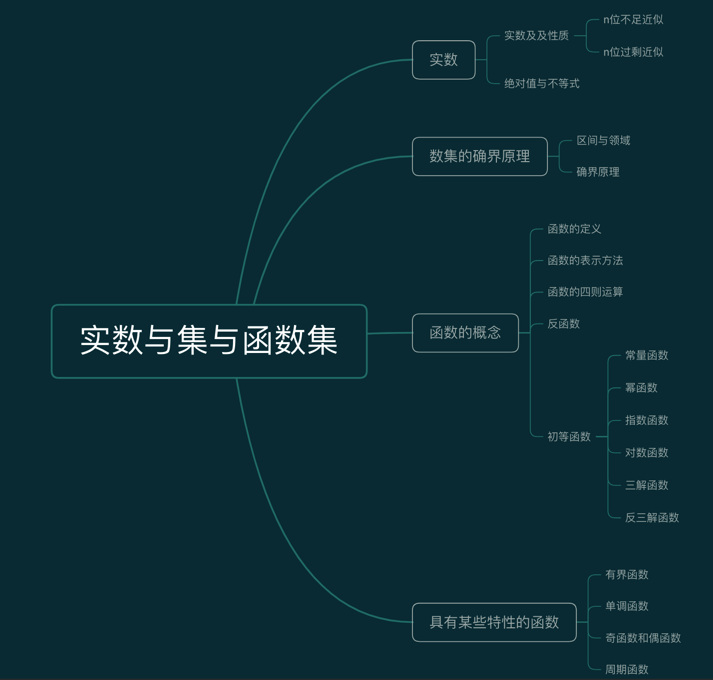# 实数集与函数## 实数

• 有理数：可以用分数形式p/q（p,q为整数，q!=0）来表示。也可以用有限十进制小数或者无限十进制循环小数来表示。

• 无理数：无限十进不循环小数为无理数。

• n位不足近似与n位过剩近似

eg: 设x,y为实属，x<y，证明：存在有理数r满足：

x<r<y


## 实数的性质

• 实数的四则运算，加减乘除（除数不为0）是封闭的，也就是运算结果仍然是实数；
• 实数集是有序的，也就是ab两个实九必须满足a=b,或者a>b,或者a<b；
• 实数的大小具有传递性，也就是a>b,b>c那么a>c
• 实数具有阿基米德性，也就是任意的实数a,b，如果b>a>0，那么存在整数n使得na>b;
• 实数集具有稠密性，也就是说任意的两个实数间，一定存在另外一个实数，这个实数可以是有理数，也可以是无理数；
• 实数集与数轴成映射关系，也就是所有的实数都在在数轴上找到对应的位置。

eg：设a,b属于实数集，证明：若对任何正数ε,有a<b+ε,则a<=b

## 绝对值与不等式

$|a| =\begin{cases} a,a>=0\ -a,a<0 \end{cases}$

• 实数的绝对值性质
1. $|a|$=$|-a|$>=0;当且仅当a=0时有$|a|$ = 0
2. -$|a|$<=a<=$|a|$
3. $|a|$<h $\iff$